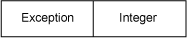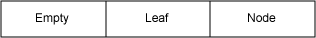通过实例学习Either 树和模式匹配

更新时间：2019年06月12日 14:36:42   作者：Neal Ford我要评论`Either<Exception, Integer> result = RomanNumeralParser.parseNumber("XLII");`

Scala 模式匹配

Scala 的众多出色功能之一就是能够使用 模式匹配 进行调度（参阅 参考资料）。与描述相比，演示更简单一些，因此我们会考虑清单 1 中的函数，将数字分数转换为字母分数：

```val VALID_GRADES = Set("A", "B", "C", "D", "F")
def letterGrade(value: Any) : String = value match {
case x:Int if (90 to 100).contains(x) => "A"
case x:Int if (80 to 90).contains(x) => "B"
case x:Int if (70 to 80).contains(x) => "C"
case x:Int if (60 to 70).contains(x) => "D"
case x:Int if (0 to 60).contains(x) => "F"
case x:String if VALID_GRADES(x.toUpperCase) => x.toUpperCase
}
printf("Sam never showed for class, scored %d, and received %s\n",

```class Color(val red:Int, val green:Int, val blue:Int)
case class Red(r:Int) extends Color(r, 0, 0)
case class Green(g:Int) extends Color(0, g, 0)
case class Blue(b:Int) extends Color(0, 0, b)
def printColor(c:Color) = c match {
case Red(v) => println("Red: " + v)
case Green(v) => println("Green: " + v)
case Blue(v) => println("Blue: " + v)
case col:Color => {
print("R: " + col.red + ", ")
print("G: " + col.green + ", ")
println("B: " + col.blue)
}
case null => println("invalid color")
}```

Java 没有提供模式匹配，因此它无法复制 Scala 的创建清晰可读的调度代码的能力。但是，通过结合使用泛型数据结构和众所周知的数据结构，可以实现更加接近的能力，这又将我带回了 Either。

Either 树

Empty 单元中不包含任何值
Leaf 单元中拥有一个特殊数据类型值
Node 指向其他 叶 或 节点```import fj.data.Either;
import static fj.data.Either.left;
import static fj.data.Either.right;
public abstract class Tree {
private Tree() {}
public abstract Either<Empty, Either<Leaf, Node>> toEither();
public static final class Empty extends Tree {
public Either<Empty, Either<Leaf, Node>> toEither() {
return left(this);
}
public Empty() {}
}
public static final class Leaf extends Tree {
public final int n;
public Either<Empty, Either<Leaf, Node>> toEither() {
return right(Either.<Leaf, Node>left(this));
}
public Leaf(int n) { this.n = n; }
}
public static final class Node extends Tree {
public final Tree left;
public final Tree right;
public Either<Empty, Either<Leaf, Node>> toEither() {
return right(Either.<Leaf, Node>right(this));
}
public Node(Tree left, Tree right) {
this.left = left;
this.right = right;
}
}
}```

Scala 的模式匹配鼓励您思考离散情况。Functional Java 的 Either 实现中的 left() 和 right() 方法都实现了 Iterable 接口；这允许我编写支持模式匹配的代码来确定树的深度，如清单 4 所示：

```static public int depth(Tree t) {
for (Empty e : t.toEither().left())
return 0;
for (Either<Leaf, Node> ln: t.toEither().right()) {
for (Leaf leaf : ln.left())
return 1;
for (Node node : ln.right())
return 1 + max(depth(node.left), depth(node.right));
}
throw new RuntimeException("Inexhaustible pattern match on tree");
}```

```static public boolean inTree(Tree t, int value) {
for (Empty e : t.toEither().left())
return false;
for (Either<Leaf, Node> ln: t.toEither().right()) {
for (Leaf leaf : ln.left())
return value == leaf.n;
for (Node node : ln.right())
return inTree(node.left, value) | inTree(node.right, value);
}
return false;
}```

```@Test
public void more_elaborate_searchp_test() {
Tree t = new Node(new Node(new Node(new Node(
new Node(new Leaf(4),new Empty()),
new Leaf(12)), new Leaf(55)),
new Empty()), new Leaf(4));
assertTrue(inTree(t, 55));
assertTrue(inTree(t, 4));
assertTrue(inTree(t, 12));
assertFalse(inTree(t, 42));
}```

```static public int occurrencesIn(Tree t, int value) {
for (Empty e: t.toEither().left())
return 0;
for (Either<Leaf, Node> ln: t.toEither().right()) {
for (Leaf leaf : ln.left())
if (value == leaf.n) return 1;
for (Node node : ln.right())
return occurrencesIn(node.left, value) + occurrencesIn(node.right, value);
}
return 0;
}```

```@Test
public void multi_branch_tree_test() {
Tree t = new Node(new Node(new Node(new Leaf(4),
new Node(new Leaf(1), new Node(
new Node(new Node(new Node(
new Node(new Node(new Leaf(10), new Leaf(0)),
new Leaf(22)), new Node(new Node(
new Node(new Leaf(4), new Empty()),
new Leaf(101)), new Leaf(555))),
new Leaf(201)), new Leaf(1000)),
new Leaf(4)))),
new Leaf(12)), new Leaf(27));
assertEquals(12, depth(t));
assertTrue(inTree(t, 555));
assertEquals(3, occurrencesIn(t, 4));
}```